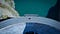Pursuing Masters in Machine Learning.

# Introduction to Logistic Regression

Moving from Linear to Logistic function.Photo by Raphaël Biscaldi on Unsplash

In the previous blog, we learned about Linear regression which we used to predict the continuous target variable. But what if the target is discrete? In this case, we use the model called Logistic regression. It a modelling algorithm that is used in the classification type of problem.

Let us clear continuous and discrete meaning with the below representation.

# Assumptions of Linear Regression

Understanding these assumptions is important.

Linear models are parametric in nature which means there are some assumptions related to such models which we need to validate before using these models. To understand these assumptions better, it is advised to get familiar with the Linear regression concepts, we have covered Linear regression with codes in detail in the previous blog. In this one, we will focus on the Assumptions of the Linear model. It is important to validate these assumptions to make a robust linear model. The linear models fail to give a good result if these assumptions are violated. This…

# Introduction to Linear Regression

## A gentle introduction to the algorithm and code for it.

This is one of the first models we get introduced to when we start our machine learning journey. It is a very simple yet elegant model to predict the continuous target variable. Before jumping into the algorithm, let's try to understand what the term regression means? It is a statistical method to establish a relationship between independent variables (input) and the dependent variable (target). It is widely used in forecasting and hypothesis testing.

Linear regression is a method to model the linear relationship between the dependent variables and independent variables. …